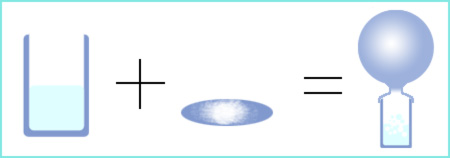Knowledge database: Basic laws of chemistry: Law of conservation of mass The law of conservation of mass is one of the basic laws of chemistry, and says that no changes can be observed in the total mass of all substances involved in a chemical reaction. This means that in a closed system, the mass of all reactants that enter a chemical reaction is equal to the total mass of products that were the result of that chemical reaction. However, today we know that this isn't completely true, because in reactions that release a large amount of energy, a small reduction in mass is present. That was not known at the time when the law was discovered because the mass loss is very small, but today this phenomenon is well known thanks to Einstein's theory of relativity, which says that mass that is lost is equal to the energy that is released, divided by the squared speed of light ( m = E/c2 ). As an example we can mention the ignition of 5 grams of phosphorus. In the mentioned reaction, thermal energy of 1.2x105 J is developed. Using the above mentioned formula, when one divides this energy with the squared speed of light, the result is the mass that is lost - only 1.35x10-9 grams, which is a very small mass. In almost all cases which chemists normally face, this kind of mass loss is negligible, so the law of conservation of mass applies regardless of this small deviation.On the picture one can see a reaction between vinegar (in the beaker) and baking soda (on the watching glass). The mass of the reactants (vinegar and baking soda), will be equal to the mass of the products. A balloon is placed over the beaker because one of the products is a gas, so the mass of this gas must also be taken into account.